×
Get Full Access to Physics - 4 Edition - Chapter 3 - Problem 40
Get Full Access to Physics - 4 Edition - Chapter 3 - Problem 40

×

# Solved: What are the direction and magnitude of your totalISBN: 9780321611116 152

## Solution for problem 40 Chapter 3

Physics | 4th Edition

• Textbook Solutions
• 2901 Step-by-step solutions solved by professors and subject experts
• Get 24/7 help from StudySoup virtual teaching assistantsPhysics | 4th Edition

4 5 1 381 Reviews
13
0
Problem 40

What are the direction and magnitude of your total displacement if you have traveled due west with a speed of 27 m/s for 125 s, then due south at 14 m/s for 66 s?

Step-by-Step Solution:

Q: What are the direction and magnitude of your total displacement if you have traveled due west with a speed of 27 m/s for 125 s, then due south at 14 m/s for 66 s?

Step By Step Solution

Step 1 of 2:

Speed of travel westwards: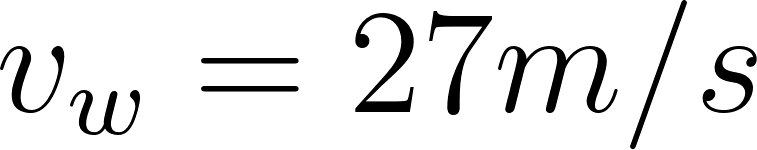Time of travel :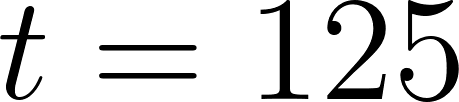Displacement:Speed of travel southwards :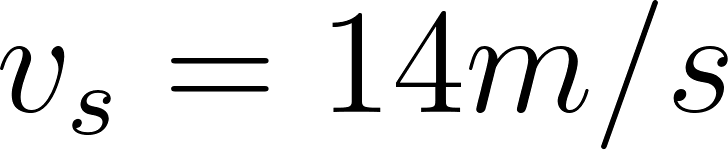Time of travel :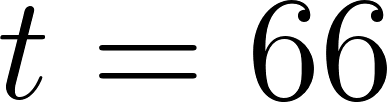Displacement: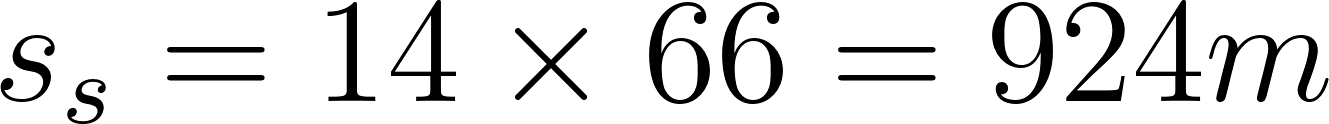Step 2 of 2

##### ISBN: 9780321611116

Physics was written by and is associated to the ISBN: 9780321611116. This textbook survival guide was created for the textbook: Physics, edition: 4. The full step-by-step solution to problem: 40 from chapter: 3 was answered by , our top Physics solution expert on 11/15/17, 04:23PM. This full solution covers the following key subjects: due, South, direction, magnitude, displacement. This expansive textbook survival guide covers 32 chapters, and 3407 solutions. Since the solution to 40 from 3 chapter was answered, more than 282 students have viewed the full step-by-step answer. The answer to “What are the direction and magnitude of your total displacement if you have traveled due west with a speed of 27 m/s for 125 s, then due south at 14 m/s for 66 s?” is broken down into a number of easy to follow steps, and 34 words.

Unlock Textbook Solution